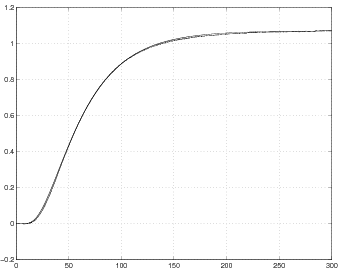]> 7.5.3 Least–square identiﬁcation using the step response

#### 7.5.3 Least–square identiﬁcation using the step response

The least–square identiﬁcation of the system parameters of the model (1.8) can be formulated as the problem of ﬁnding a quadruple $\left(a,{R}_{a},{R}_{0},{R}_{1}\right)$, which minimizes the performance index

 $\mathcal{𝒥}\left(a,{R}_{a},{R}_{0},{R}_{1}\right)=\sum _{i=1}^{M}{\left[y\left(mh\right)-{y}_{e}\left(mh\right)\right]}^{2}$ (7.29)

where $y\left(mh\right)$ stands for the theoretical value of the step response $y$ at time $mh$, $m=1,2,\dots ,M$, evaluated by means of formulae (7.26) $÷$ (7.28), and ${y}_{e}\left(mh\right)$, is a practical value of the step response at time $mh$, $m=1,2,\dots ,M$, obtained from measurements; $h$ denotes the time discretization step, and $M$ is the ﬁnal horizon of observation expressed by the total number of trials. In experiments we have assumed $h=0.1$, $M=3000$. The unknown coefficient ${y}_{0}$ is determined from the requirement that the steady–state values of $y$ and ${y}_{e}$ coincide. To be more precise, we have

${lim}_{t\to \infty }{y}_{e}\left(t\right)={y}_{0}{lim}_{t\to \infty }{y}_{1}\left(t\right)=-{y}_{0}{〈c,{\mathcal{𝒜}}^{-1}b〉}_{\text{H}}=-{y}_{0}\sum _{n=1}^{\infty }\frac{1}{{\lambda }_{n}}{〈b,{e}_{n}〉}_{\text{H}}{〈{e}_{n},c〉}_{\text{H}}.$

Assuming that the horizon of observation is sufficiently large we may take

${y}_{e}\left(Mh\right)={y}_{0}{y}_{1}\left(Mh\right).$

In numerical calculations we used truncation of series (7.26) to $25$ terms, which seems to be a reasonable compromise between the time of calculations and the accuracy. The optimal values of $a$, ${R}_{a}$, ${R}_{0}$ and ${R}_{1}$ were determined by means of the procedure fmins from the Matlab/Optimization Toolbox package. Since the performance index $\mathcal{𝒥}$ is not unimodal the procedure fmins of the optimization without constraints was used several times and with various starting points. Final results are presented in Table 7.1.

 Table 7.1: The optimal values of the heat exchange coefficients
 $a$ ${R}_{a}$ ${R}_{0}$ ${R}_{1}$ $\mathcal{𝒥}$ 0.000945 0.02709999 0 0 0.0877040968

The experimental and theoretical step responses are depicted in Figure 7.1.Figure 7.1: Comparison of the experimental and the optimal theoretical step responses